## Figures index

#### Gowthaman S, Nasvi MCM

American Journal of Civil Engineering and Architecture. 2017, 5(5), 187-195 doi:10.12691/ajcea-5-5-2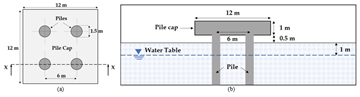• Figure 1. (a) Plan-view of 2 × 2 square pile group, and (b) Sectional view X-X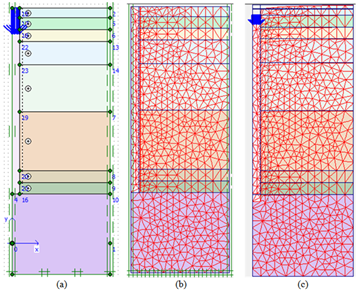• Figure 2. (a) Geometry of the Finite Element Model (b) FE mesh and (c) deformed mesh for complete LE analysis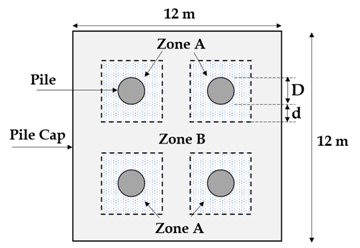• Figure 3. Pile group layout of combined analysis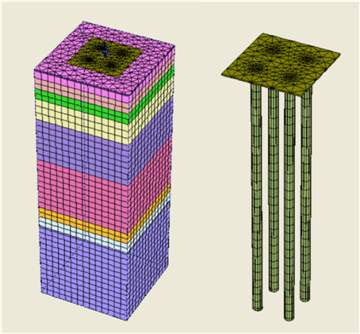• Figure 4. Meshed geometry of group pile foundation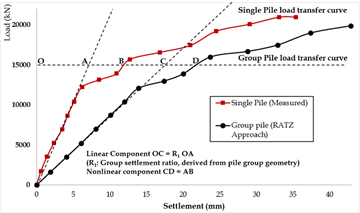• Figure 5. Load-settlement curve of the pile group obtained from RATZ approach• Figure 6. Comparison of load-settlement plots obtained from FEA and field test result for single pile analysis• Figure 7. Comparison of load-settlement plots obtained from FEA and field test result for a single pile using corrected modulus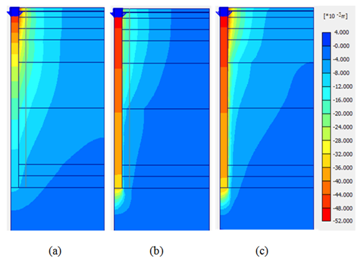• Figure 8. Settlement contours of soil obtained when it was modeled as complete (a) LE model, (b) MC model and (c) HS model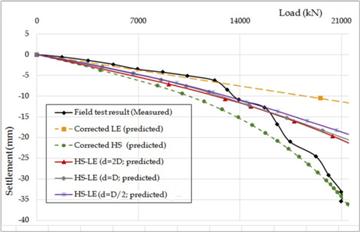• Figure 9. Comparison of load-settlement behaviour obtained from HS-LE analysis and measured Field Test result• Figure 10. Comparison of load-settlement behaviour obtained from HS-MC analysis and measured Field Test result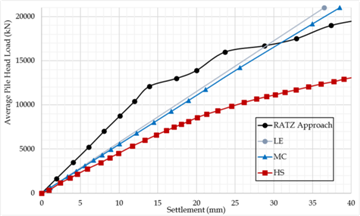• Figure 11. Comparison of load settlement behaviour of pile group from linear and non-linear FE analysis and RATZ analytical approach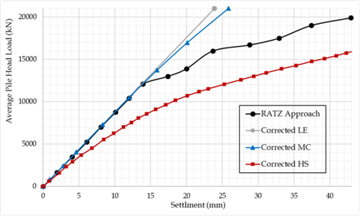• Figure 12. Comparison of load settlement behaviour of pile group from linear and non-linear FE analysis with corrected modulus and RATZ analytical approach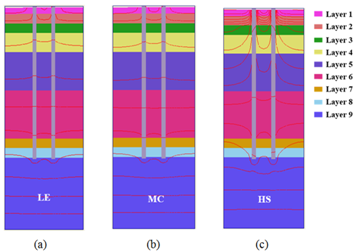• Figure 13. Settlement contours of the considered pile group from (a) LE, (b) MC and (c) HS analysis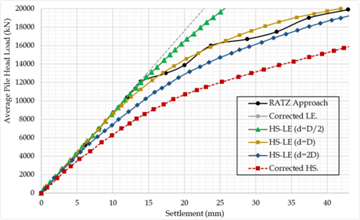• Figure 14. Comparison of load settlement behaviour of pile group from HS-LE analysis and RATZ analytical approach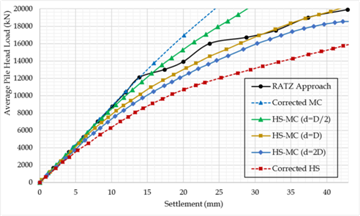• Figure 15. Comparison of load settlement behaviour of pile group from HS-LE analysis and RATZ analytical approach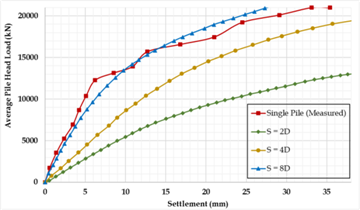• Figure 16. Load-settlement relationships of pile group with different spacing• Figure 17. Vertical deformation contours of pile group with the spacing of (a) 2D and (b) 8D